# Example: Workflow in R

This example provides a schematic workflow for processing vector and raster data in R.

## Get raster data

Firstly, we import some raster data into our working environment Therefore, we need to load a package to handle raster data in R, preferable `terra`. To get some example data we will use the package `geodata` If the packages are not available, we need to install it first with `install.packages("terra")`.

``````library(terra)
``````

We now can use the function `getData()` to download some raster data: In this example a global map of precipitation values at 10 minutes spatial resolution.Set a path to a temporary folder where you want to store the downloaded data.

``````prec <- geodata::worldclim_global(var="prec", res=10, path="D:/temp")
``````

``````##class       : SpatRaster
##dimensions  : 1080, 2160, 12  (nrow, ncol, nlyr)
##resolution  : 0.1666667, 0.1666667  (x, y)
##extent      : -180, 180, -90, 90  (xmin, xmax, ymin, ymax)
##coord. ref. : lon/lat WGS 84 (EPSG:4326)
##sources     : wc2.1_10m_prec_01.tif
##              wc2.1_10m_prec_02.tif
##              wc2.1_10m_prec_03.tif
##              ... and 9 more source(s)
##names       : wc2.1~ec_01, wc2.1~ec_02, wc2.1~ec_03, wc2.1~ec_04, wc2.1~ec_05, wc2.1~ec_06, ...
##min values  :           0,           0,           0,           0,           0,           0, ...
##max values  :         908,         793,         720,        1004,        2068,        2210, ...
``````

… and can be quickly and simply visualized with `terra::plot()`. Note that the object type is a `SpatRaster` with 12 layers, one for each month of the year.

``````terra::plot(prec\$wc2.1_10m_prec_01)
``````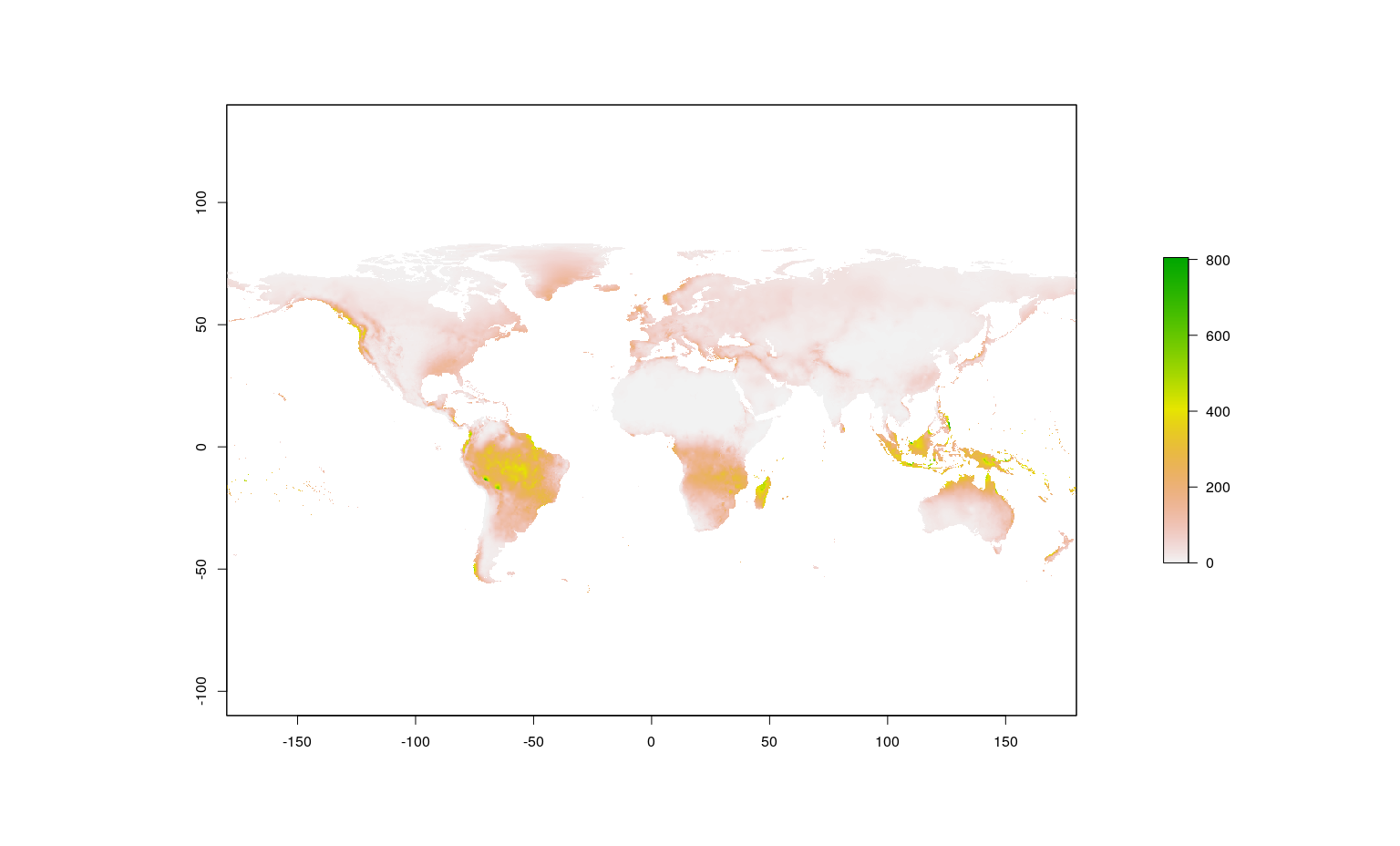## Get vector data

Secondly, we add some vector data to our working environment. For example the administrative boundaries of France at the country level:

``````fra <- geodata::gadm(country='FRA', level=0, path="D:/temp")
``````

``````## class       : SpatVector
## geometry    : polygons
## dimensions  : 1, 2  (geometries, attributes)
## extent      : -5.143751, 9.560416, 41.33375, 51.0894  (xmin, xmax, ymin, ymax)
## coord. ref. : lon/lat WGS 84 (EPSG:4326)
## names       : GID_0 COUNTRY
## type        : <chr>   <chr>
## values      :   FRA  France
``````

Note that the object type here is a `SpatVector` (defined in package `terra`) with one feature (i.e. with a single polygon), a certain extent (which can also be extracted with `terra::ext(fra)`), a CRS (which can be extracted with `terra::crs(fra)`), and two variables with some values (in this case country abbreviation and name).

Also vector data can quickly and simply be visualized with `plot()`

``````plot(fra)
``````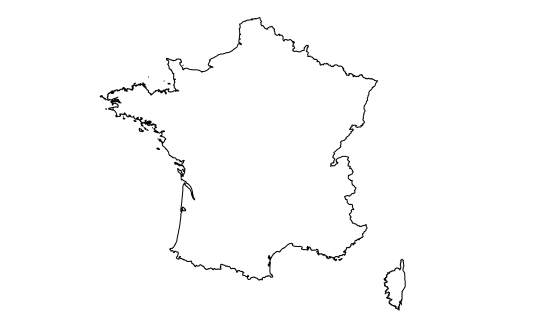## Set extent

We can use the extent of one spatial object to crop (i.e. to cut out) another spatial object. In this example, we will crop the raster map(s) with the extent defined in the vector object. This is going to work because both objects have the same CRS. Note that `crop()` processes all layers of the input raster stack.

``````cropped_prec <- crop(prec, ext(fra))
``````

For function arguments see `?crop()`. Now we have precipitation maps of France:

``````plot(cropped_prec\$wc2.1_10m_prec_01)
``````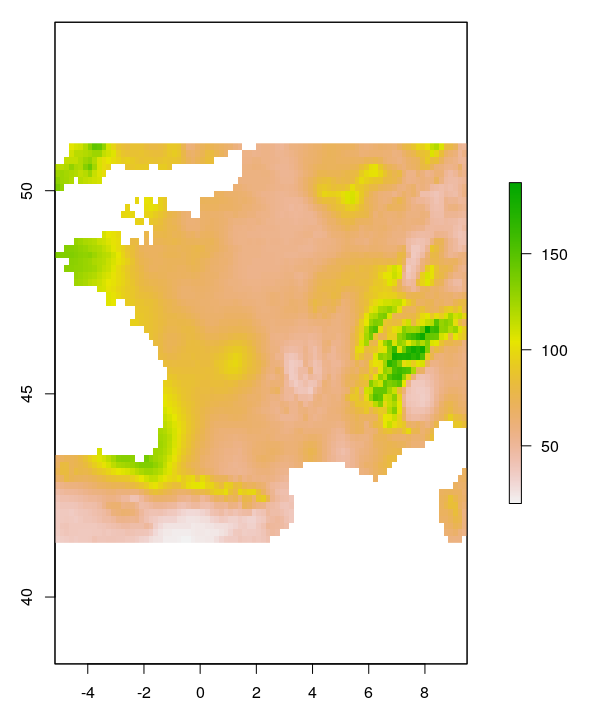## Vector operations

A simple vector operation would be to clip a spatial object not by the extent of another spatial object but by features or polygons of any shape.

We will now use the country boundary of France for clipping of the already cropped precipitation maps with the `mask()` function of the raster package:

``````clipped_prec <- mask(cropped_prec, fra)
``````

The result is a `SpatRaster` object with 12 layers, one for each month of the year.

Again, the result can quickly and simply be visualized with `plot()`

``````plot(clipped_prec\$wc2.1_10m_prec_01)
``````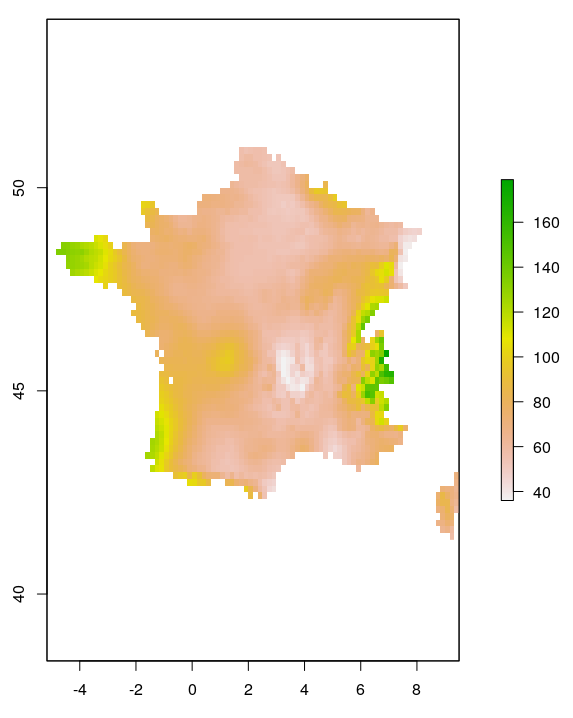## Raster operations

The created SpatRaster now contains precipitation values of France on a monthly basis. What if we want to have a single precipitation layer with annual precipitation values? We would need to sum up the values of all 12 precipitation layers for each pixel location. This can be done by:

``````clipped_prec_sum <- sum(clipped_prec)
``````

The resulting raster map looks like this:

``````plot(clipped_prec_sum)
``````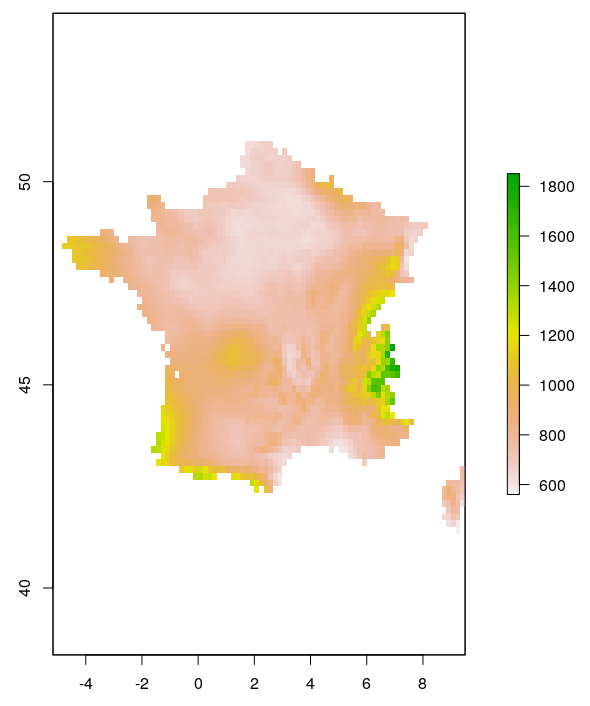Note the different value range, which now stands for the annual amount of precipitation (in mm).

## Mapping

We now combine all the above created spatial objects to create a single simple map:

``````plot(clipped_prec_sum)
points(2.349014, 48.864716, pch=8, cex=2) # roughly the location of Paris
``````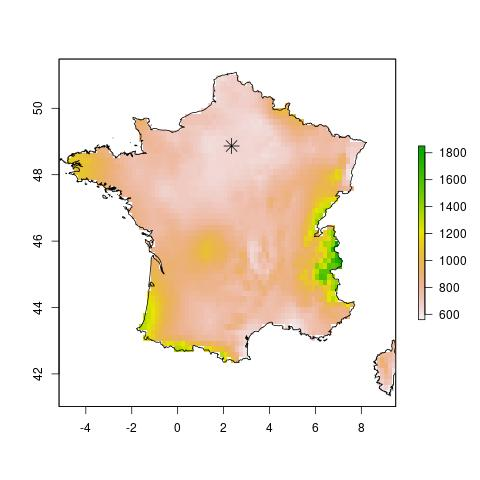## Write out

The above created overlay of maps can be written to file as an ordinary image with e.g.

``````jpeg("FirstSimpleMap.jpg")
plot(clipped_prec_sum)
points(2.349014, 48.864716, pch=8, cex=2)
dev.off()
``````

Note that this is a raster image without geographic information. If you want to write out the spatial objects with geographic information, use e.g. `terra::writeRaster()` or `raster::writeVector()`.

## Other important functions

• Reading in raster data from file: `terra::rast()`
• Reading in vector data from file: `terra::vect()`